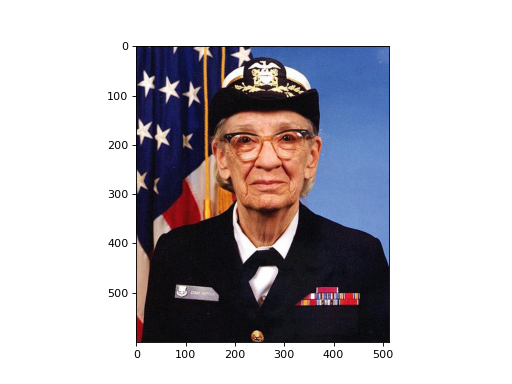# User Guide¶

By importing this package, you can use Bob native array reading and writing routines to load and save files using various image formats, using the simple plug-in technology for `bob.io.base`, i.e., `bob.io.base.load()` and `bob.io.base.save()`.

```>>> import bob.io.base
>>> import bob.io.image  # under the hood: loads Bob plugin for image files
```

In the following example, an image generated randomly using the method NumPy `numpy.random.random_integers()`, is saved in lossless PNG format. The image must be of type `uint8` or `uint16`:

```>>> my_image = numpy.random.random_integers(0,255,(3,256,256))
>>> bob.io.base.save(my_image.astype('uint8'), 'testimage.png') # saving the image in png format
>>> assert (my_image_copy == my_image).all()
```

The loaded image files can be 3D arrays (for RGB format) or 2D arrays (for greyscale) of type `uint8` or `uint16`.

You can also get information about images without loading them using `bob.io.base.peek()`:

```>>> bob.io.base.peek(path_to_image)
(dtype('uint8'), (3, 600, 512), (307200, 512, 1))
```

In order to visualize the loaded image you can use `bob.io.image.imshow()`:

```>>> img = bob.io.base.load(path_to_image)
>>> bob.io.image.imshow(img)
```Or you can just get a view (not copy) of your image that is `matplotlib.pyplot` compatible using `bob.io.image.to_matplotlib()`:

```>>> img_view_for_matplotlib = bob.io.image.to_matplotlib(img)
>>> assert img_view_for_matplotlib.shape[-1] == 3
>>> assert img_view_for_matplotlib.base is img
```

You can also get the original image back using `bob.io.image.to_bob()`. This function works with images, batches of images, videos, and higher dimensional arrays that contain images. Moreover, see `bob.io.image.opencvbgr_to_bob` and `bob.io.image.bob_to_opencvbgr`.